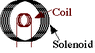# Mutual Inductance of solenoid and coil

• hellogirl88

#### hellogirl88

Find the mutual inductance (H) of the solenoid and coil shown below. The solenoid has 5263 turns per meter and an area 112 cm^2, and the coil has 230 turns and an area 19 cm^2.

I know that mutual inductance = (N1 Φ1)/ I2 but I have no idea where to begin since they don't provide the current or flux. How can I find those?

Thanks!

#### Attachments

•coils.gif
929 bytes · Views: 553
Hi hellogirl88,

Find the mutual inductance (H) of the solenoid and coil shown below. The solenoid has 5263 turns per meter and an area 112 cm^2, and the coil has 230 turns and an area 19 cm^2.

I know that mutual inductance = (N1 Φ1)/ I2 but I have no idea where to begin since they don't provide the current or flux. How can I find those?

Thanks!

To use the mutual inductance formula, you assume that one of the objects (either the coil or the solenoid) has some current I, and then calculate the flux that is prodcued in the other object. (The answer will be the same no matter which one you use for the current, but the calculation is much easier for one than the other.)

(By the way, the diagram makes it look like the solenoid is short. I'm assuming that it is just supposed to show a small part of it, and the solenoid is really long.)